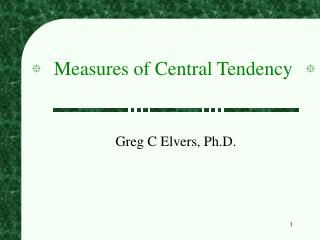DownloadDownload PresentationMeasures of Central Tendency

# Measures of Central Tendency

Download Presentation## Measures of Central Tendency

- - - - - - - - - - - - - - - - - - - - - - - - - - - E N D - - - - - - - - - - - - - - - - - - - - - - - - - - -
##### Presentation Transcript

1. Measures of Central Tendency Greg C Elvers, Ph.D.

2. Measures of Central Tendency • A measure of central tendency is a descriptive statistic that describes the average, or typical value of a set of scores • There are three common measures of central tendency: • the mode • the median • the mean

3. The Mode • The mode is the score that occurs most frequently in a set of data

4. Bimodal Distributions • When a distribution has two “modes,” it is called bimodal

5. Multimodal Distributions • If a distribution has more than 2 “modes,” it is called multimodal

6. When To Use the Mode • The mode is not a very useful measure of central tendency • It is insensitive to large changes in the data set • That is, two data sets that are very different from each other can have the same mode

7. When To Use the Mode • The mode is primarily used with nominally scaled data • It is the only measure of central tendency that is appropriate for nominally scaled data

8. The Median • The median is simply another name for the 50th percentile • It is the score in the middle; half of the scores are larger than the median and half of the scores are smaller than the median

9. How To Calculate the Median • Conceptually, it is easy to calculate the median • There are many minor problems that can occur; it is best to let a computer do it • Sort the data from highest to lowest • Find the score in the middle • middle = (N + 1) / 2 • If N, the number of scores, is even the median is the average of the middle two scores

10. Median Example • What is the median of the following scores:10 8 14 15 7 3 3 8 12 10 9 • Sort the scores:15 14 12 10 10 9 8 8 7 3 3 • Determine the middle score:middle = (N + 1) / 2 = (11 + 1) / 2 = 6 • Middle score = median = 9

11. Median Example • What is the median of the following scores:24 18 19 42 16 12 • Sort the scores:42 24 19 18 16 12 • Determine the middle score:middle = (N + 1) / 2 = (6 + 1) / 2 = 3.5 • Median = average of 3rd and 4th scores:(19 + 18) / 2 = 18.5

12. When To Use the Median • The median is often used when the distribution of scores is either positively or negatively skewed • The few really large scores (positively skewed) or really small scores (negatively skewed) will not overly influence the median

13. The Mean • The mean is: • the arithmetic average of all the scores(X)/N • the number, m, that makes (X - m) equal to 0 • the number, m, that makes (X - m)2 a minimum • The mean of a population is represented by the Greek letter ; the mean of a sample is represented by X

14. Calculating the Mean • Calculate the mean of the following data:1 5 4 3 2 • Sum the scores (X):1 + 5 + 4 + 3 + 2 = 15 • Divide the sum (X = 15) by the number of scores (N = 5):15 / 5 = 3 • Mean = X = 3

15. When To Use the Mean • You should use the mean when • the data are interval or ratio scaled • Many people will use the mean with ordinally scaled data too • and the data are not skewed • The mean is preferred because it is sensitive to every score • If you change one score in the data set, the mean will change

16. Relations Between the Measures of Central Tendency • In symmetrical distributions, the median and mean are equal • For normal distributions, mean = median = mode • In positively skewed distributions, the mean is greater than the median • In negatively skewed distributions, the mean is smaller than the median